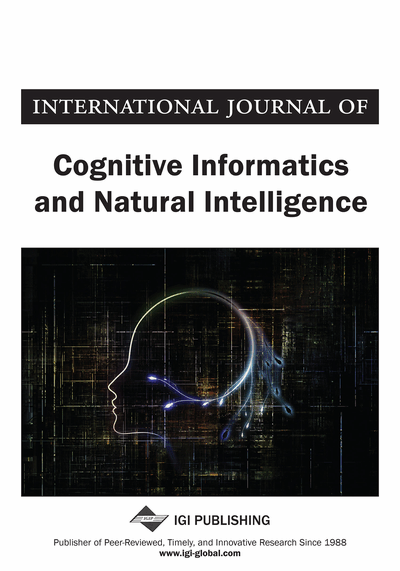# Towards Developing the Piece-Wise Linear Neural Network Algorithm for Rule Extraction

Veronica Chan (University of Regina, Regina, Canada) and Christine Chan (University of Regina, Faculty of Engineering and Applied Science, Regina, Canada)
DOI: 10.4018/IJCINI.2017040104
Available
\$37.50
No Current Special Offers

## Abstract

This paper discusses development and application of a decomposition neural network rule extraction algorithm for nonlinear regression problems. The algorithm is called the piece-wise linear artificial neural network or PWL-ANN algorithm. The objective of the algorithm is to “open up” the black box of a neural network model so that rules in the form of linear equations are generated by approximating the sigmoid activation functions of the hidden neurons in an artificial neural network (ANN). The preliminary results showed that the algorithm gives high fidelity and satisfactory results on sixteen of the nineteen tested datasets. By analyzing the values of R2 given by the PWL approximation on the hidden neurons and the overall output, it is evident that in addition to accurate approximation of each individual node of a given ANN model, there are more factors affecting the fidelity of the PWL-ANN algorithm Nevertheless, the algorithm shows promising potential for domains when better understanding about the problem is needed.
Article Preview
Top

## Introduction

Artificial neural network (ANN) is a popular data mining approach, in a wide range of area of study (Xu & Zang, 2008; Yaremchuk & Dawson, 2008), because of its high classification or predictive accuracy, and resistance to noise. However, in addition to high prediction accuracy, researchers often need to gain better understanding of the problems at hand (Chan, 2007), and ANNs are black boxes that cannot be interpreted. To overcome this deficiency, the rule extraction approach can be adopted so as to generate explicit information from the analysis results of the trained ANNs. The objective of this study is to develop a decompositional neural network rule extraction algorithm for non-linear regression problems. The approach adopted is to model a given dataset using the ANN approach and the originally trained neural network is assumed to be a three-layer feed-forward backpropagation neural network with a sigmoid activation function. Since these are the most common types of neural network models, this will be the target models on which rule extraction will be performed. Also, based on experience, one hidden layer in an ANN is typically sufficient to solve most non-linear problems without overfitting. Although the pedagogical type of rule extraction algorithms is better in terms of computational complexity and generality than decompositional algorithms, the decompositional approach is the focus because the objective is to explore the trained neural network and “open the black box”. The algorithm approximates the activation functions of a given ANN model with piece-wise linear (PWL) equations and generates explicit information in the form of numerical formulae. The targeted problems are regression problems related to engineering domains. In terms of expressive power, we would like to generate “rules” expressed as linear numeric functions in the form of

• Y = a1x1 + a2x2+… + b

Since the research objective is to understand the working mechanism of the trained neural network model, fidelity to the trained ANN will be the primary evaluation criterion of the developed algorithm. This paper is organized as follows: Section 2 discusses the motivation of the work based on observations derived from some previous works, and some background literature related to research work on ANN rule extraction algorithms. Section 3 describes the proposed methodology and Section 4 presents analysis of some preliminary results. Section 5 gives some directions for future work and Section 6 is the conclusions.

Top

## Background Literature And Motivation

There are three approaches to ANN rule extraction: (1) decompositional, which extracts rules by examining the activation and weights of the hidden layer neurons; (2) pedagogical, which extracts rules by mapping the relationships between the inputs and outputs as closely as possible to those given by the trained ANN model without opening up the “black-box” of the ANN models; and (3) eclectic, which is a hybrid of the two previous approaches. Most studies on ANN rule extraction focus on classification problems (Augasta & Kathirvalavakumar, 2012), when in reality many problems encountered in the real-world contexts are regression problems. In classification problems, the output variables are class labels, whereas in regression problems, the output variables are continuous values.

Rule extraction algorithms for ANN can be classified into three approaches based on the criterion of translucency of the algorithm: decompositional, pedagogical and eclectic (Andrews et al., 1995) The approach of decompositional algorithms aims to extract rules by examining activation functions and weights of the hidden layer neurons, and this type of algorithms are considered to be completely translucent. On the other end of the translucency spectrum is the pedagogical approach, which extracts rules by mapping the relationship between the inputs and outputs as closely as possible to that given by the trained ANN model without exploring the ANN models. The underlying ANN models are still viewed as a “black-box” and “translucency” is not a priority. The eclectic approach is a hybrid of the other two approaches and lies in the middle on the translucency spectrum.

## Complete Article List

Search this Journal:
Reset
Volume 16: 1 Issue (2022): Forthcoming, Available for Pre-Order
Volume 15: 4 Issues (2021)
Volume 14: 4 Issues (2020)
Volume 13: 4 Issues (2019)
Volume 12: 4 Issues (2018)
Volume 11: 4 Issues (2017)
Volume 10: 4 Issues (2016)
Volume 9: 4 Issues (2015)
Volume 8: 4 Issues (2014)
Volume 7: 4 Issues (2013)
Volume 6: 4 Issues (2012)
Volume 5: 4 Issues (2011)
Volume 4: 4 Issues (2010)
Volume 3: 4 Issues (2009)
Volume 2: 4 Issues (2008)
Volume 1: 4 Issues (2007)
View Complete Journal Contents Listing